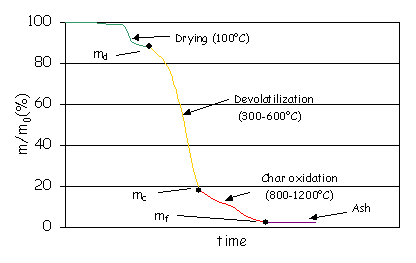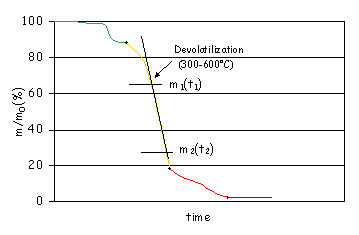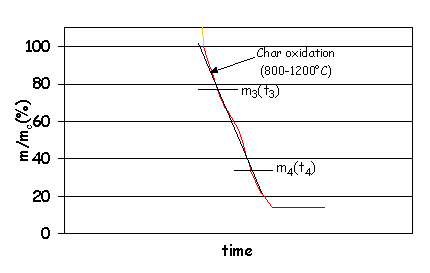• ### How do I make a basic combustion characterisation of biofuel?

Date posted:

• Post Author

### 1. General

The general
concept of fuel characterisation is outlined in Combustion
File 48
. The following describes the procedures to undertake a “first
order” or basic combustion characterisation. A biofuel is primarily described
by its [GLOSS]proximate analysis[/GLOSS] and [GLOSS]ultimate analysis[/GLOSS].
The proximate analysis can be obtained by means of [GLOSS]thermogravimetric analysis[/GLOSS] (TGA). From the ultimate analysis the
[GLOSS]calorific value[/GLOSS] of the fuel can be calculated. This technique can also give
information about the rate of [GLOSS]devolatilisation[/GLOSS] and the rate of [GLOSS]char oxidation[/GLOSS].

### 2. Thermogravimetric Analysis (TGA)

The
instrument measures the weight of a biofuel sample inside an oven under
controlled temperature. Figure 1 shows how the sample weight decreases as the
temperature rises in the oven. The sample first dries and then looses its
volatile components ([GLOSS]devolatilisation[/GLOSS] or [GLOSS]pyrolysis[/GLOSS])
at around 300-600°C. The solid residue after devolatilisation is called char
and contains mainly carbon (fixed carbon) and ash. The char oxidises at 800-1200°C
in presence of oxygen.Figure 1: Result of the thermogravimetric analysis.

### 3. Proximate Analysis

The proximate
analysis tells us about the moisture content of the fuel, the amount of volatile
components, the fixed carbon and the ash content. The data are usually presented
on dry basis.

• Moisture content (%) = 100 – md

• Volatile matter (%, moisture free (mf)*)
= (md– mc)/ md x 100

• Ash content (%, mf) = mf/md
x 100

• Fixed carbon (%, mf) = 100 – volatile matter
(%, mf) – ash content (%, mf)

The weight loss during devolatilisation
depends on the temperature and heating rate and therefore this additional
information is usually given.

*Often
expresses as “dry”.

### 4. Ultimate Analysis and Calorific Value

This
analysis gives the elemental composition of the biofuel, usually in moisture and
ash free basis (dry ash free-[GLOSS]daf[/GLOSS]). The
basic components are carbon, hydrogen, oxygen, nitrogen and a negligible amount
of sulphur.

The
[GLOSS]higher calorific value[/GLOSS]
(HCV) can be calculated from the ultimate analysis as:

HCV
(MJ/kg dry fuel)= (34.1 C + 102 H + 6.3 N + 19.1 S – 9.85 O)/100

where
C, H, N, S and O represent the weight(%, mf) of carbon, hydrogen, nitrogen,
sulphur and oxygen in the fuel.

The
[GLOSS]higher calorific value[/GLOSS]
can also be
measured in a bomb calorimeter by reacting the fuel with oxygen and measuring
the heat released to a known quantity of water.

The
[GLOSS]effective calorific value[/GLOSS]
(EHV) is also useful, and for wood fuels is expressed as:

EHV
(kWh/kg wet fuel)= 5.32 – 6.01 (moisture content (%))

EHV
(kWh/kg dry fuel)= 5.32 – 0.69 (moisture content (%))/(1-moisture
content (%))

### 5. Rate of Devolatilisation

It is necessary in
many situations to quantify how reactive is the fuel during devolatilisation.
The devolatilisation rate is the slope of the TGA curve, as shown in Figure 2.

Devolatilisation
rate (%/min) = (m1(%)-m2(%))/(t2(min)-t1(min)).Figure
2: Devolatilisation rate.

### 6. Rate of Char Oxidation

This value quantifies the reactivity of
the solid char during the combustion. In this case, the mass of char is not
referred to the initial mass of fuel, m0, but to the initial mass of
char, mc.

Char oxidation
rate (%/min)= (m3(%)-m4(%))/(t4(min)-t3(min)).Figure
3: Char oxidation rate.

### You may also like

• #### What are the principles behind the use of multi-directional probes for velocity measurements in furnaces?

12th November 2001

• #### What is a kiln?

19th September 2002